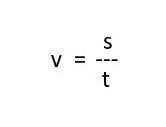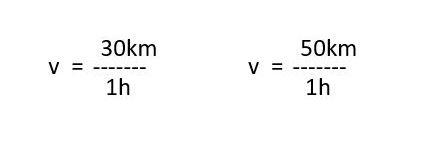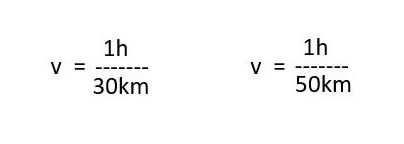# I am still standing## In a physics forum it was about representing speed.

v = s/t.

Velocity is the distance travelled divided by time elapsed. (v – speed; s – distance; t – time).

The higher the value (v), the faster the distance travelled.30 km in 1h is slower than 50 km in 1h – 50 km per hour means a higher speed.

## Since one participant in this discussion made a mistake with the formula and interchanged s and t, he did not represent the speed.

### The reverse would be the time spent while travelling: v = t/s.

Here v is the time consumed for the distance travelled.

The higher this value (v), the slower the distance was covered.1/30 = 0.033333… is greater than 1/50 = 0.02 and this first path-time represents a slower speed as the second.

For an object at rest (distance travelled = 0) this means a division by ZERO and this is not defined.

Surely, this means that you should always be in motion, otherwise you don’t exist or mathematically you are simply infinity like a black hole!

In the example above, you can use 30km/0h, but it is physically wrong. Because you cannot travel from a to b in ZERO time. You always need a time. Since the speed of light is the fastest speed, there is no need to think about it: it is simply not physically feasible. In the example below, however, you can use ZERO. Because you can sit, lie or stand for an hour.

Funny thing, mathematics sometimes. Isn’t it?### Average Rating

5 Star
0%
4 Star
0%
3 Star
0%
2 Star
0%
1 Star
0%

#### Most Commented

[email-subscribers-form id=”1″]

#### Featured Videos